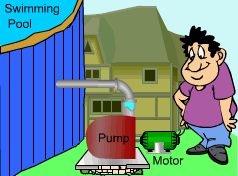Ch 6. Entropy Multimedia Engineering Thermodynamics Entropy Tds Relations EntropyChange IsentropicProcess IsentropicEfficiency EntropyBalance (1) EntropyBalance (2) ReversibleWork
 Chapter 1. Basics 2. Pure Substances 3. First Law 4. Energy Analysis 5. Second Law 6. Entropy 7. Exergy Analysis 8. Gas Power Cyc 9. Brayton Cycle 10. Rankine Cycle Appendix Basic Math Units Thermo Tables Search eBooks Dynamics Fluids Math Mechanics Statics Thermodynamics Author(s): Meirong Huang Kurt Gramoll ©Kurt GramollTHERMODYNAMICS - CASE STUDY IntroductionProblem Description Mr. Williams has a small swimming pool in his backyard. The pump that pumps water from a well to the pool is broken. However, he found a used pump in a second-hand electric utility store. To insure that the pump matches his motor, he needs to figure out the power required to run the pump. What is known: The pump is capable of increasing the pressure of water up to 300 kPa. The flow rate through the pump is 20 kg/min. The pump has an isentropic efficiency of 85%. Question What is the required power input to the pump? Approach Take the pump as a control volume. Model the pump as an adiabatic pump. Use the definition of isentropic efficiency of pump to calculate the enthalpy change of the process. Use the energy balance to calculate the power input to the pump.